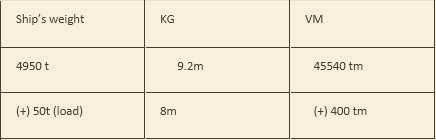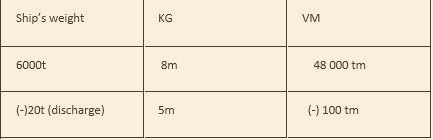top of pageSearch

# Chapter 6

### 1. In a vessel of 8800 tonnes displacement and KG 6.2m, 200 tonnes of cargo was loaded in the lower hold 1.7m above the keel. Find the final KG.

Solution:

Given:-

Weight = 8800t, Original KG =6.2m, Cargo loaded = 200t, KG = 1.7mFinal W – 9000 t Final VM 54900 tm

Final KG = (Final VM/ Final W) Final KG = (54900 / 9000) = 6.1m

Here ‘VM’ stands for vertical moments.

### 2. 600 tonnes of cargo were discharged from a vessel from the upper 11m above the keel. In the original KG displacement were 6m and 12600 tonnes, calculate the KG.

Solution:-

Given:- Weight =12600 t Original KG =6m Cargo discharged = 600 t,

Cargo discharge 11 m above the keelFinal Weight =12000 t Final VM =69000tm

Final KG = (Final VM / Final Weight)

Final KG = ( 69,000 / 12000)

= 5.75m

### Solution:Final W 10000t Final VM =41000tm

Final KG = (Final VM / Final Weight) Final KG = ( 41000 / 10000 ) = 4.11m.

### 4. 500 tonnes of cargo of discharge from the lower the lower hold (KG 3m ) of a vessel whose displacement and KG before discharging were 11500 tonnes and 6.3m . Find the final KG.

Solution:

Given:-

Weight = 11500t Initial KG = 6.3m Cargo discharged = 500t, KG =3mFinal W =11000t Final VM =70950tm

Final KG = (Final VM / Final Weight) Final KG = (70,950/11000) =6.45m

### 5. 500 tonnes of cargo was shifted 15 meters vertically downwards in a vessel of 10000 tonnes displacement. Find the effect it has on the KG of the vessel and whether KG increases or decreases.

Solution:

Given:-

Weight =10000t, Cargo shifted downward =500t,

GG1() = (w x d)/( total weight) = (500 x 15)/ 10000 =0.75m

Since, The weight is shifted vertically downward, the KG of ship will also go down parallel to the KG of the weight

Hence, KG of the ship decrease by 0.75m

### 6. In a vessel of 9000 tonnes displacement , KG 10.5m, 300 tonnes of cargo was shifted from the LH (KG 2.5m)to the UDC (KG 11.5m). Find the resultant KG of teh vessel.

Solution :

Weight =9000 t Original KG = 10.5m , Cargo shifted = 300 t

Vertical Distance of cargo shifted = (height of UD – height of LH) = (11.5 -2.5) = 9m upwards.Final W = 9000 Final VM =200t

Final KG = (Final VM / Final Weight) Final KG = (97200 / 9,000) = 10.8m.

### 7. In a vessel of 9009 tones displacement, KG 8.7m, how many tonnes of cargo can be loaded on the upper deck (KG 15m ) so that the final KG would be comes 9m.

Solution:-

Given:- Weight = 9009 t, Original KG =8.7m,

Let cargo loaded be X tonnes Final KG =9mFinal W = (9009 + X) t Final VM =(78378.3 + 15X) tm

Final KG = (Final VM / Final Weight) Final KG = (78378.3 +15X ) /(9009 + X) 9.0 = (78378.3 + 15X)/ (9009+X) 9 x (9009+X) = (78378.3 + 15X) (81081 + 9X) = (15X + 78378.3) Hence, 6X = 78378 .3 – 81081 6X = 2702.7t X = 450.45 t

### b. After shifting is over.

Solution:

Given: Weight = 12000t, Initial KG =7.6m, Cargo shifted = 300t, Change of KG = (8 – 2) = 6m downwards

Derrick Height = 20m, KG of the Weight = 8m

(since the weight is on the UD)

Case – 1Final W =12000 t Final VM =94800tm

Final KG = (Final VM / Final Weight) FKG = (94800 / 12000) = 7.9m

Case – 2Final W =12000 Final VM = 89400tm

Final KG = (Final VM / Final Weight) Final KG = (89400 / 12000) = 7.45m.

### a. when the weight is hanging by the derrick on the centre line but 2m above the UD , and

Solution:

Given:- Weight = 4950t, Initial KG = 9.2m Cargo loaded = 50t , UD KG =8m Height of the derrick = 25m

Case – 1Final W = 5000t FVM = 46790tm

Final KG = (Final VM / Final Weight) Final KG = (46790 / 5000) = 9.358m

Case – 2Final W = 5000t Final VM = 45940tm

Final KG = (Final VM / Final Weight) Final KG = (45940 / 5000) = 9.188m

### b. After discharging .

Solution:-

Given:- Weight = 6000t, Original KG = 8m, Cargo discharged = 20t, KG of cargo discharged = 5m Height of the derrick = 22m

Case – 1Final W = 6000 Final VM 48340 tm

Final KG = (Final VM / Final Weight) Final KG = (48340 / 6,000) = 8.056m

Case – 2Final W =5980t Final VM = 47900 tm

Final KG = (Final VM / Final Weight) Final KG = (47900/5980) = 8.010m.

10,505 views1 comment

See All
bottom of page